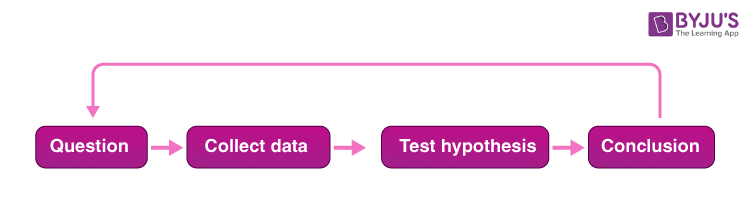# Scientific Methods

## What is Scientific Method?

The Scientific method is a process with the help of which scientists try to investigate, verify, or construct an accurate and reliable version of any natural phenomena. They are done by creating an objective framework for the purpose of scientific inquiry and analysing the results scientifically to come to a conclusion that either supports or contradicts the observation made at the beginning.

## Scientific Method Steps

The aim of all scientific methods is the same, that is, to analyse the observation made at the beginning. Still, various steps are adopted per the requirement of any given observation. However, there is a generally accepted sequence of steps in scientific methods.1. Observation and formulation of a question: This is the first step of a scientific method. To start one, an observation has to be made into any observable aspect or phenomena of the universe, and a question needs to be asked about that aspect. For example, you can ask, “Why is the sky black at night? or “Why is air invisible?”
1. Data Collection and Hypothesis: The next step involved in the scientific method is to collect all related data and formulate a hypothesis based on the observation. The hypothesis could be the cause of the phenomena, its effect, or its relation to any other phenomena.
1. Testing the hypothesis: After the hypothesis is made, it needs to be tested scientifically. Scientists do this by conducting experiments. The aim of these experiments is to determine whether the hypothesis agrees with or contradicts the observations made in the real world. The confidence in the hypothesis increases or decreases based on the result of the experiments.
1. Analysis and Conclusion: This step involves the use of proper mathematical and other scientific procedures to determine the results of the experiment. Based on the analysis, the future course of action can be determined. If the data found in the analysis is consistent with the hypothesis, it is accepted. If not, then it is rejected or modified and analysed again.

It must be remembered that a hypothesis cannot be proved or disproved by doing one experiment. It needs to be done repeatedly until there are no discrepancies in the data and the result. When there are no discrepancies and the hypothesis is proved, it is accepted as a ‘theory’.

### Scientific Method Examples

Following is an example of the scientific method:

Growing bean plants:

1. What is the purpose: The main purpose of this experiment is to know where the bean plant should be kept inside or outside to check the growth rate and also set the time frame as four weeks.
2. Construction of hypothesis: The hypothesis used is that the bean plant can grow anywhere if the scientific methods are used.
3. Executing the hypothesis and collecting the data: Four bean plants are planted in identical pots using the same soil. Two are placed inside, and the other two are placed outside. Parameters like the amount of exposure to sunlight, and amount of water all are the same. After the completion of four weeks, all four plant sizes are measured.
4. Analyse the data: While analysing the data, the average height of plants should be taken into account from both places to determine which environment is more suitable for growing the bean plants.
5. Conclusion: The conclusion is drawn after analyzing the data.
6. Results: Results can be reported in the form of a tabular form.

## Frequently Asked Questions – FAQs

Q1

### What is scientific method?

The Scientific method is a process with the help of which scientists try to investigate, verify, or construct an accurate and reliable version of any natural phenomena. They are done by creating an objective framework for the purpose of scientific inquiry and analysing the results scientifically to come to a conclusion that either supports or contradicts the observation made at the beginning.
Q2

### What is hypothesis?

A hypothesis is an assumption that is made based on some evidence. This is the initial point of any investigation that translates the research questions into predictions. It includes components like variables, population and the relation between the variables. A research hypothesis is a hypothesis that is used to test the relationship between two or more variables.
Q3

### Give an example of a simple hypothesis.

Consumption of sugary drinks daily results in obesity. This is an example of a simple hypothesis.
Q4

### Define complex hypothesis.

A complex hypothesis shows the relationship between two or more dependent variables and two or more independent variables.
Q5

### What are the steps of the scientific method?

Observation, data collection, testing and analysing.
Q6

### What is the aim of scientific methods?

The aim of all the scientific methods is to analyze the observation made at the beginning but there are various steps adopted as per the requirement of any given observation.
Q7

FALSE
Q8

### Explain the step: Analysis and Conclusion.

This step involves the use of proper mathematical and other scientific procedures to determine the results of the experiment. Based on the analysis, the future course of action can be determined. If the data found in the analysis is consistent with the hypothesis, it is accepted. If not, then it is rejected or modified and analyzed again.